# OECD Tourism flows data analysis 7 - regression analysis with infer package of RUnsplashAlexander Cifuentesが撮影した写真

This post is following of above post.

In this post, I will do cross sectional regression analysis using infer package.

Fisrt, I select one year for cross sectional regression. I check which year has the most number of observations.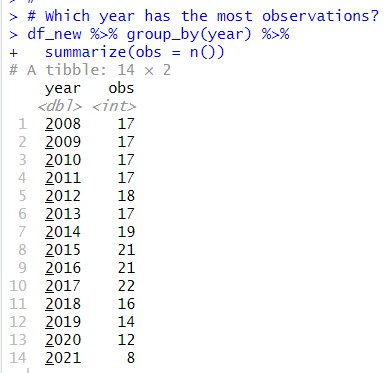2017 year has the most observations.

So, I make a data frame which contains only 2017.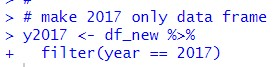Let's see summary statistics of y2017.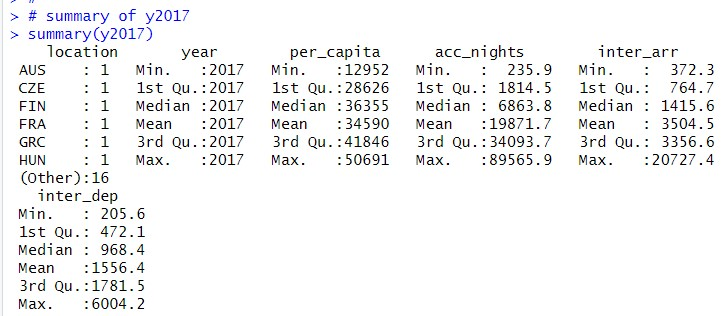Let's see scatter plots.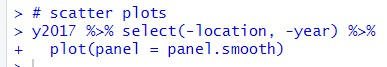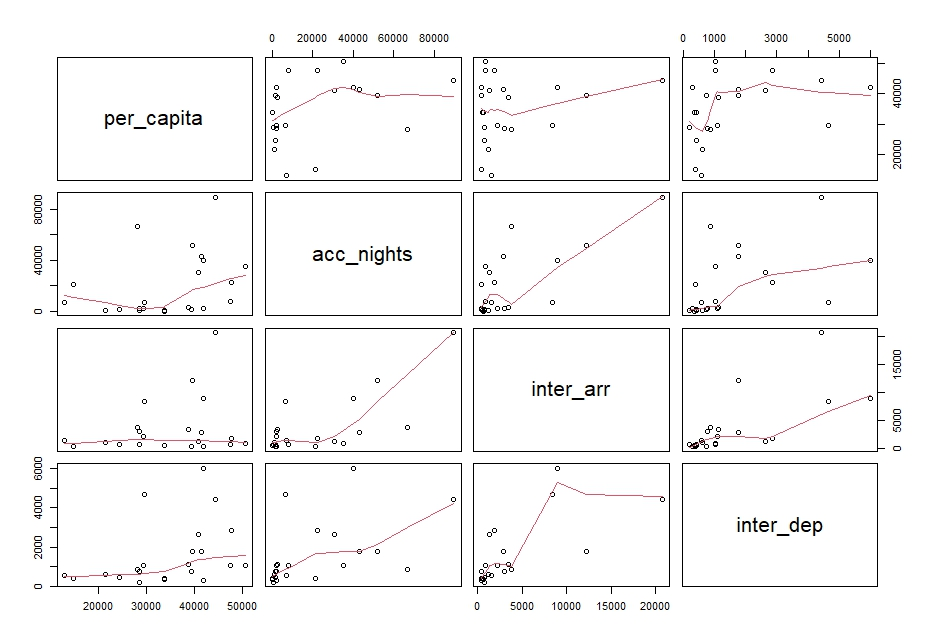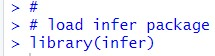In this post, I will analysis per_capita ~ acc_nights + inter_arr + inter_dep model with traditional lm() function eco system and infer package eco system.

Fisrt, I make the both regression results.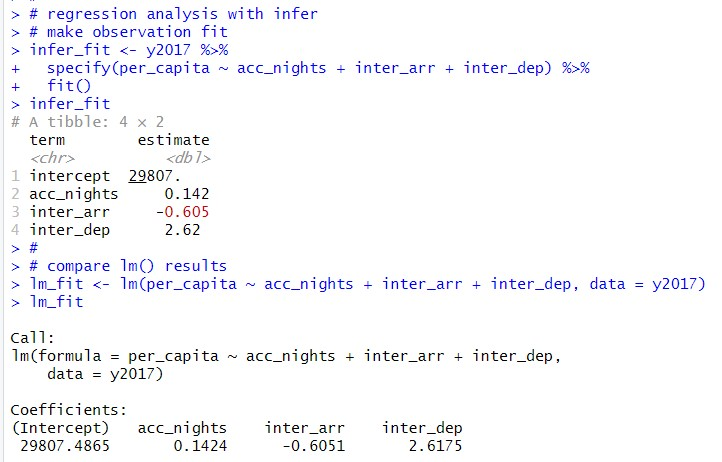the both results gets the same slope estimates.

acc_nights is 0.142,

inter_arr is -0.605

inter_dep is 2.62.

To get confidence intervals with infer ecosystem, I need to make null distribution of slope estimates.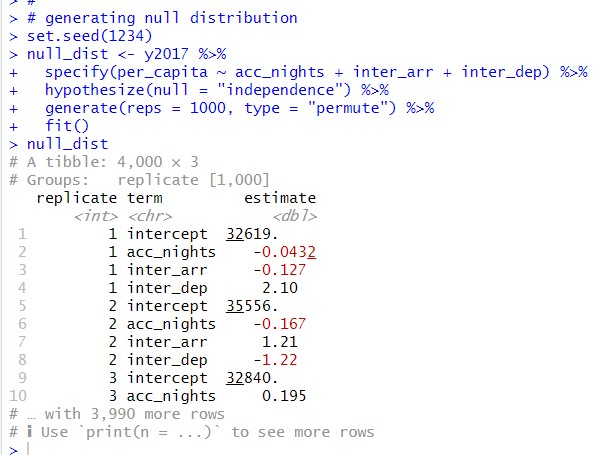Then, I use get_confidence_interval() function.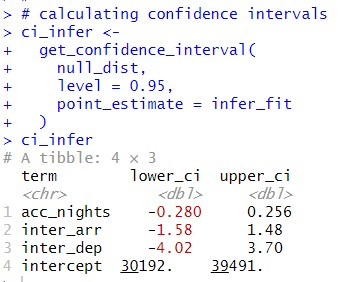In lm() ecosystem, I can use confint() function to get confidence intervals.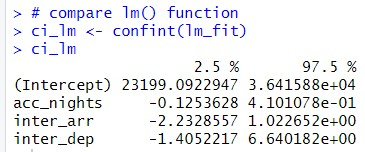All right, let's visualize confidence intervals.

First, acc_nights confidence intervals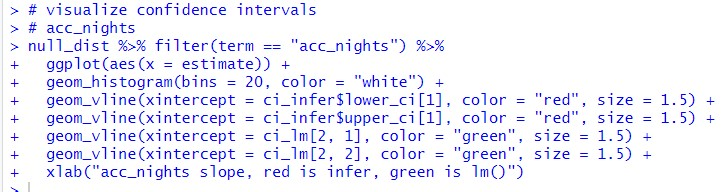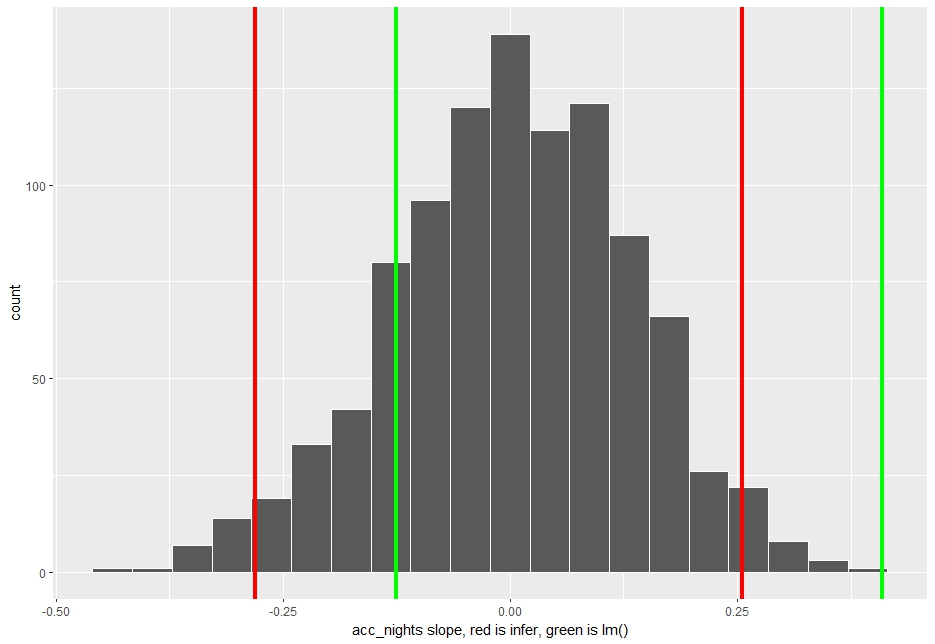The both confident intervals include zero, so, acc_nights is not statistically significant.

Next, inter_arr.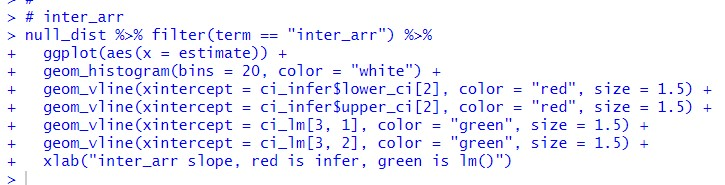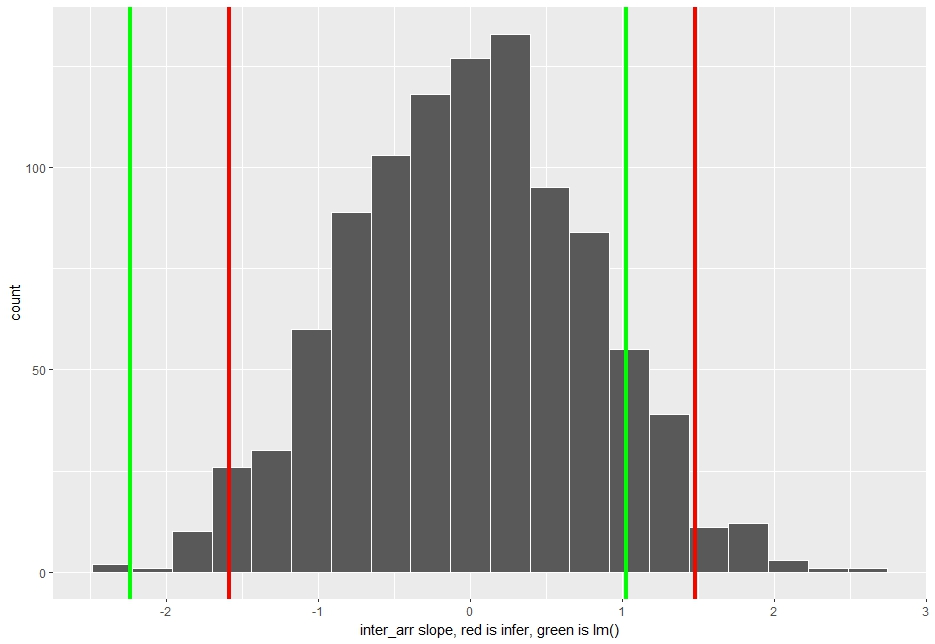Again, the both confidence intervals include zero, so that inter_arr is not statistically significant.

Last, inter_dep.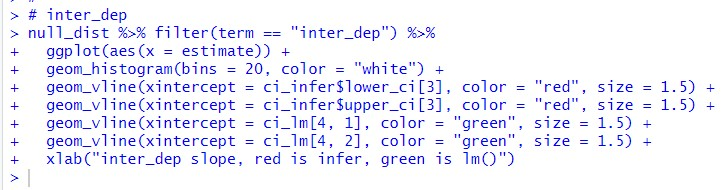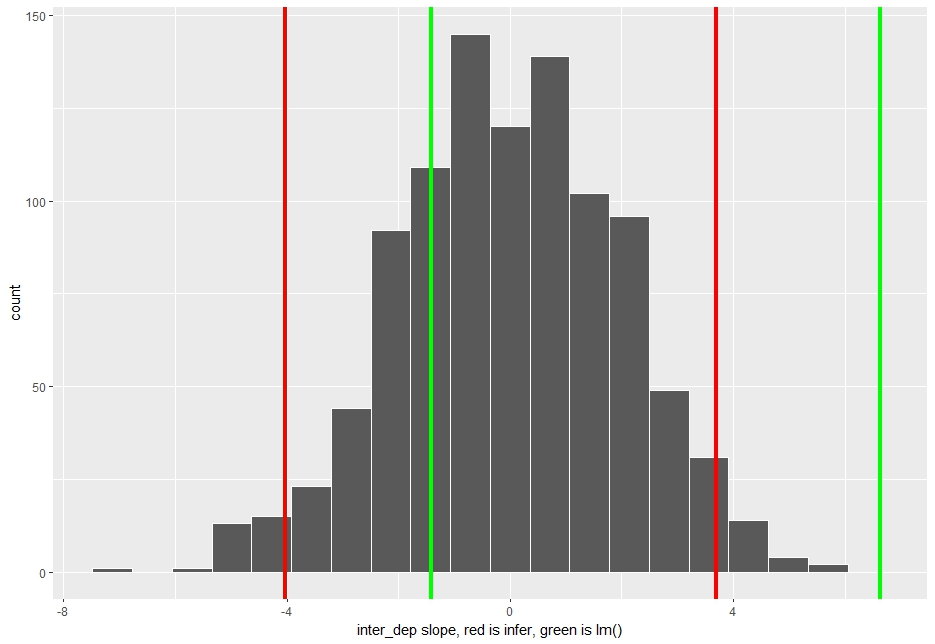The both inter_dep slope estimate confidence intervals contain zero, so inter_dep is not statistically significant.

That's it. Thank you!

To read from the 1st post,